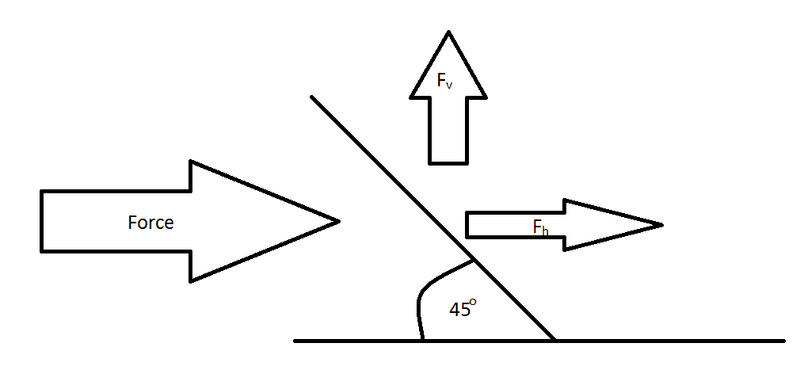# Distribution of force at different angles

## Homework Statement

If a force (wind) hits a plane which is at a 45 degree angle, how is that force distributed? How much is translated vertically and how much is translated horizontally? My guess is that 50% is translated vertically and 50% is translated horizontally. Here is an image to clarify:And from there. How do you calculate the distribution at any angle, say 15 degrees?

## Homework Equations

I am guessing it is some kind of trig.

## The Attempt at a Solution

Unsure how to proceed. I have never been taught anything about this.

## Answers and Replies

tiny-tim
Science Advisor
Homework Helper
welcome to pf!

hi memoguy! welcome to pf!If a force (wind) hits a plane which is at a 45 degree angle, how is that force distributed? How much is translated vertically and how much is translated horizontally? My guess is that 50% is translated vertically and 50% is translated horizontally.

if you're supposed to treat wind like any other force, then yes, 50:50And from there. How do you calculate the distribution at any angle, say 15 degrees?

I am guessing it is some kind of trig.

for any other force, you can "resolve" the force into "components" in two perpendicular directions …

one is times cos, the other is times sin(but i'm not sure that wind behaves like that …

a lot of momentum and energy is taken away by the wind itself changing direction when it hits the surface)​

CWatters
Science Advisor
Homework Helper
Gold Member
Wind does not have a built in force that can be divided like that. For example there is no equation that says..

Vertical Force (aka "Lift") * Horizontal force (aka "Drag") = a constant

What you have is a flat plate wing section. That will produce lift and drag forces that depend on the angle of attack, wind speed and other factors.

Read...
http://en.wikipedia.org/wiki/Lift_coefficient
http://en.wikipedia.org/wiki/Drag_coefficient

The Coefficient of Lift (CL) and drag (CD) for flat plate sections of various aspect ratios are illustrated on page 10 ...

http://cwrowley.princeton.edu/papers/Taira_reno07.pdf

which suggests for 45 degrees and infinite aspect ratio..

CL is about 1.4
CD is about 1.5

To calculate the actual force plug numbers into the equations at wikipedia or better still write an equation for the ratio of the lift to drag force. See what cancels.

SteamKing
Staff Emeritus
Science Advisor
Homework Helper
New trig values: cos 45 = sin 45 = 0.5?

Ok, so you can't do it via that trig.

To calculate the actual force plug numbers into the equations at wikipedia or better still write an equation for the ratio of the lift to drag force. See what cancels.

Well when you divide the lift coefficient by the drag coefficient like so:
(l/(0.5*p*v^2*a))/((2f)/(p*v^2*a))

It simplifies to l/f which doesn't really get me anywhere. The lift/drag coefficient doesn't take angle of attack into account. I would have to have very specific values. All I have is power output of a wind turbine at different angles of attack.

------------------

Do you think it would be valid to simply discuss newtons third law VS drag. By this I mean that when air hits the airofoil it is deflected downward. This pushes the blade upward. As the AOA is increased the surface area directly exposed to the air is increased. This results in a greater deflection of air downward and therefore a greater upward force, as per newtons third law of motion. However, as AOA is increased drag is also increased, once the angle becomes two high, high amounts of drag are produced, decreasing the power output.

However, I still think that the force distribution is important. I just don't know how to proceed, I don't have the data to satisfy any of the equations I have found.

To make matters worse the blade seems to behave in a way that is not consistent with any data found on the internet. It is most effective when the angle of attack is +/- 75 degrees!

CWatters
Science Advisor
Homework Helper
Gold Member
Check that ratio. I think you will find that it works out to..

FL/FD = CL/CD

where..

FL is the lift force
FD is the drag force

The angle of attack is taken into account because it is included in the Coefficients of Lift and Drag.

To make matters worse the blade seems to behave in a way that is not consistent with any data found on the internet. It is most effective when the angle of attack is +/- 75 degrees!

Ah now i remember, this is the little wind mill problem right?

Working out what's going on is complicated because the AOA is not the same as the blade angle to the shaft. As the blade starts to move the Angle of Attack changes due to the "Apparent Wind". When held stationary a 75 degree blade angle would mean the blade is stalled, however as the rotor accelerates the real AOA to the airflow reduce to the point that the rotor unstalls...

http://ecorenovator.org/wind-power-tutorial/